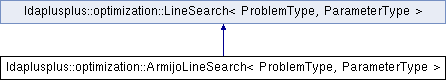LDA++
ldaplusplus::optimization::ArmijoLineSearch< ProblemType, ParameterType > Class Template Reference

#include <GradientDescent.hpp>

Inheritance diagram for ldaplusplus::optimization::ArmijoLineSearch< ProblemType, ParameterType >:## Public Types

typedef ParameterType::Scalar ScalarPublic Types inherited from ldaplusplus::optimization::LineSearch< ProblemType, ParameterType >
typedef ParameterType::Scalar Scalar

## Public Member Functions

ArmijoLineSearch (Scalar beta=0.001, Scalar tau=0.5)

Scalar search (const ProblemType &problem, Eigen::Ref< ParameterType > x0, const ParameterType &grad_x0, const ParameterType &direction)

## Detailed Description

### template<typename ProblemType, typename ParameterType> class ldaplusplus::optimization::ArmijoLineSearch< ProblemType, ParameterType >

Armijo line search is a simple backtracking line search where the Armijo condition is required.

The Armijo condition is the following if $$p_k$$ is the negative direction and $$g_k$$ the gradient. In Armijo line search we search the largest $$a_k \in \{\tau^n \mid n \in \{0\} \cup \mathbb{N}\}$$ for which the Armijo condition stands.

$f(x_k - a_k p_k) \leq f(x_k) - a_k b g_k^T p_k$

$$f(x_k) - a_k b g_k^T p_k$$ is a linear approximation of the function at $$x_k$$ (scaled by $$b$$) that we assume to be the upper bound for the decrease.

In all the above $$p_k$$ is assumed to be of unit length.

See Wolfe Conditions.

## Constructor & Destructor Documentation

template<typename ProblemType , typename ParameterType >
 ldaplusplus::optimization::ArmijoLineSearch< ProblemType, ParameterType >::ArmijoLineSearch ( Scalar beta = 0.001, Scalar tau = 0.5 )
inline
Parameters
 beta The amount of scaling to do the linear decrease tau Defines the set of $$a_k$$ to try in the line search

## Member Function Documentation

template<typename ProblemType , typename ParameterType >
 Scalar ldaplusplus::optimization::ArmijoLineSearch< ProblemType, ParameterType >::search ( const ProblemType & problem, Eigen::Ref< ParameterType > x0, const ParameterType & grad_x0, const ParameterType & direction )
inlinevirtual

Search for a good enough function value in the direction given and the function given.

This function changes its parameter x0 which is passed by reference.

Parameters
 problem The function to be minimized x0 The improved position (passed by reference) grad_x0 The gradient at the initial x0 direction The direction of search which can be different than the gradient to account for Newton methods
Returns
The function value at the final x0

The documentation for this class was generated from the following file: